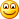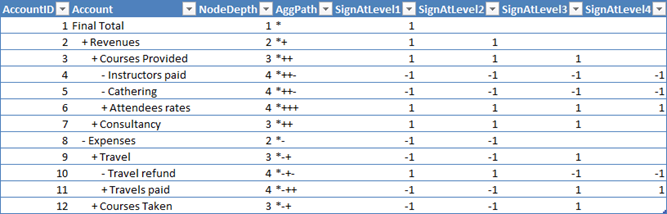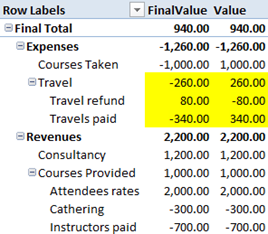Following my last post about parent/child hierarchies in PowerPivot, I worked a bit more to implement a very useful feature of Parent/Child hierarchies in SSAS which is obviously missing in PowerPivot, i.e. unary operators. A unary operator is simply the aggregation function that needs to be used to aggregate values of children over their parent.

Unary operators are very useful in accountings where you might have incomes and expenses in the same hierarchy and, at the total level, you want to subtract expenses from incomes, in order to get a meaningful total. Clearly, you might obtain a good result adding a sign in front of the values in the fact table but, doing so, you miss the opportunity to create more than one hierarchy over the same data where values might get different signs in different hierarchies. For example, I might have a hierarchy where expenses are subtracted from the total and another one, with a different structure, where the expenses need to be summed with other values. Thus, I suppose that signing the value in the fact table is not a viable solution and I want to put the signs into the hierarchy.

Implementing unary operators with the four mathematical operations is not easy at all. It can be done, but with a quite big effort and some severe limitations that I am not going to describe here. Moreover, in my personal experience I have never used neither multiplication nor division in any hierarchy, I have always used sum and subtraction only. Thus, I am going to focus on these two basic operations only, leaving the four operations to a next post, if somebody asks me to.Let us start with the table structure for the demo. We have an Accounts table, which holds the hierarchy, and a Transactions table with the transactions. All amounts in the transactions table are positive numbers.

`CREATE TABLE Accounts (     AccountId         INT,      Account           VARCHAR (100),     ParentAccountId   INT,     Aggregator        VARCHAR(1) )  CREATE TABLE Transactions (     AccountId    INT,     Description  VARCHAR (100),     Amount       MONEY ) `

Having the two tables, we can now fill them with some data:

`INSERT INTO Accounts (AccountId, Account, ParentAccountId, Aggregator) VALUES           ( 1, 'Final Total',       NULL, ' '), -- Grand Total         ( 2, 'Revenues',             1, '+'), --   ADD Total Revenues to Grand Total         ( 3, 'Courses Provided',     2, '+'), --     SUM Courses to Revenues         ( 4, 'Instructors paid',     3, '-'), --       SUBTRACT paid instructors from Courses         ( 5, 'Cathering',            3, '-'), --     SUBTRACT cathering from Courses         ( 6, 'Attendees rates',      3, '+'), --       SUM attendee rates to Courses         ( 7, 'Consultancy',          2, '+'), --     SUM Consultancy to Revenues         ( 8, 'Expenses',             1, '-'), --   SUBTRACT Expenses to Total         ( 9, 'Travel',               8, '+'), --     SUM travel to Expenses         (10, 'Travel refund',        9, '-'), --       SUBTRACT travels refund to Travels         (11, 'Travels paid',         9, '+'), --       SUM travels paid to Expenses         (12, 'Courses Taken',        8, '+'); --     SUM courses taken to Expenses  INSERT INTO Transactions VALUES      ( 4, 'Marco Russo',         200),     ( 4, 'Rob Collie',          500),     ( 5, 'Sandwiches',          300),     ( 6, 'Course in Seattle',   800),     ( 6, 'Course in Boston',   1200),     ( 7, 'Work in Microsoft',   400),     ( 7, 'Work in Apple',       500),     ( 7, 'Work in SQL Blog',    300),     (10, 'Travel to Seattle',    80),     (11, 'Travel to Boston',    150),     (11, 'Travel to London',    190),     (12, 'SSAS Maestros',      1000);  `

Take a look at the Accounts table where I provided some descriptions for the operations. You can see, for example, that account number 4 (Instructors Paid), even if it belongs to Revenues, needs to be subtracted from there because it is, in reality, an expense. The same applies for account number 10 (Travel Refund) which belongs to an expense (Travel) but must be subtracted from there. Moreover, since Expenses needs to be subtracted from the grand total, it happens that Travel Refund needs to summed to the grand total and subtracted from expenses. As you can see, an account can change its sign during the hierarchy navigation, which is a perfectly normal and desired behavior. Nevertheless, we need a way to handle this.

You should already know, from my previous post, that the hierarchy need to be flattened in order to work with PowerPivot. I am not going to bore you again with the flattening technique, here I am focusing on the unary operators only.

The idea is that we need to compute the sign that each account should have at the different levels of the hierarchy. Let us take, as an example, the account number 10: Travel Refund. It is a leaf node in the hierarchy at level 4. Let us look at how we should aggregate its value at the various levels:

• Level 4 (itself) a minus to show that its value will be subtracted from Travel
• Level 3 (Travel) a minus since its value will be subtracted from Travel
• At level (Expenses) it its sign becomes a plus, since is is aggregated into expenses which value is then subtracted from the grand total. Having traversed two minus, its sign becomes now a plus.
• At level 1 (Final Total) it remains a plus since the grand total does not change the sign of the last aggregation (which was Expenses)

You see that at each different level of the hierarchy an account might be summed or subtracted, depending on the number of minus signs that have been encountered during the “path” from the leaf node to the final total. With this basic idea in mind, it is now clear that we need to compute the “sign path” of each node and, from there, compute the sign of the account at the different levels of the hierarchy.

This is easily accomplished with the next query:

`WITH AggPaths as (     SELECT          AccountID   = A.accountID,          Account     = CAST (A.Account AS VARCHAR (100)),         NodeDepth   = 1,         AggPath     = CAST (a.aggregator AS VARCHAR (100))      FROM          Accounts a where ParentAccountId IS NULL     UNION ALL     SELECT          AccountID   = Children.accountID,          Account     = CAST (REPLICATE (' ', Parent.NodeDepth * 4) + Children.Aggregator + ' ' + Children.Account AS VARCHAR (100)),         NodeDepth   = Parent.NodeDepth + 1,         AggPath     = CAST (Parent.AggPath + Children.aggregator AS VARCHAR (100))      FROM Accounts Children         INNER JOIN AggPaths Parent on Children.ParentAccountId = Parent.AccountID) SELECT      AggPaths.*,   SignAtLevel1 = CASE WHEN NodeDepth < 1 THEN NULL WHEN dbo.COUNTMINUS (SUBSTRING (AggPath, 1, 4)) % 2 = 0 THEN 1 ELSE -1 END,     SignAtLevel2 = CASE WHEN NodeDepth < 2 THEN NULL WHEN dbo.COUNTMINUS (SUBSTRING (AggPath, 2, 4)) % 2 = 0 THEN 1 ELSE -1 END,     SignAtLevel3 = CASE WHEN NodeDepth < 3 THEN NULL WHEN dbo.COUNTMINUS (SUBSTRING (AggPath, 3, 4)) % 2 = 0 THEN 1 ELSE -1 END,     SignAtLevel4 = CASE WHEN NodeDepth < 4 THEN NULL WHEN dbo.COUNTMINUS (SUBSTRING (AggPath, 4, 4)) % 2 = 0 THEN 1 ELSE -1 END FROM AggPaths ORDER BY AccountID `

I have used a function, CountMinus, which counts the number of minus in a string and whose definition is simply:

`CREATE FUNCTION CountMinus (@Param VARCHAR (100))  RETURNS INT AS BEGIN     RETURN LEN (@Param) - LEN (REPLACE (@Param, '-', '')); END `

The result of the query is this:You can see that the AggPath column contains the “sign path” of each account and the various SignAtLevel contain the sign that this account should have when aggregated at the various levels. At the root level I added a star to avoid having a blank, which would return incorrect values for SUBSTRING.

Using the query and the original table, JOINED together, you can easily load the data inside PowerPivot and start some analysis. Now, to show the values in a PivotTable, you can decide two different methods:

• Use, at each level, the sign that the value should have when aggregated with its parent
• Always use the sign that the node will have when shown at level 1

The difference between the two visualizations is evident in the next figure:In “Value” I used the first technique. You can see that “Travel refund” has a minus sign, indicating that it will be subtracted from expenses. The same account, on the other measure, has a plus sign, indicating that the value will be summed to the final total. Both the information are correct, it all depends on how the user is used to look at numbers. I personally prefer the visualization of “FinalValue”, since my brain recognizes it as correct, while I refuse to understand the other one. Nevertheless, it is a matter of taste, I have met plenty of accounting managers who strongly prefer the other visualization.

Now, how do we compute the two formulas? It is pretty easy, indeed. It is enough to note that, in the filter context of each cell, we are browsing the hierarchy at a defined and well known level. We simply need to sum all the amounts with a plus (in that level) and subtract all the amounts with a minus (again, in that level). Thus, the formula for “FinalValue” is:

`=IF ([ShowRow] && [CurrentLevel] > 0,         CALCULATE (SUM (Transactions[Amount]), Accounts[SignAtLevel1] = +1)        - CALCULATE (SUM (Transactions[Amount]), Accounts[SignAtLevel1] = -1),     BLANK () )`

Where I make use of a couple of measures [ShowRow] and [CurrentLevel] which return true/false depending on whether the row should be visible or not and [CurrentLevel] which computes the current level of visualization. See my previous post to learn how they can be easily defined. Apart from those two measures, the formula is simply a subtraction of two CALCULATE which impose the correct filtering on the accounts, separating them into two distinct groups: the ones that need to be summed and the ones that need to be subtracted. It might be worth noting that the CALCULATE operates in a pre-existent filter context where all and only the children of the current node are visible and the new filter added to the context does not interfere with that selection, since it operates on a different column.

The formula for “Value” is very similar, just a bit tedious to write:

`=IF ([ShowRow],     IF ([CurrentLevel] = 4,          CALCULATE (SUM (Transactions[Amount]), Accounts[SignAtLevel4] = +1)        - CALCULATE (SUM (Transactions[Amount]), Accounts[SignAtLevel4] = -1),     IF ([CurrentLevel] = 3,          CALCULATE (SUM (Transactions[Amount]), Accounts[SignAtLevel3] = +1)        - CALCULATE (SUM (Transactions[Amount]), Accounts[SignAtLevel3] = -1),     IF ([CurrentLevel] = 2,          CALCULATE (SUM (Transactions[Amount]), Accounts[SignAtLevel2] = +1)        - CALCULATE (SUM (Transactions[Amount]), Accounts[SignAtLevel2] = -1),     IF ([CurrentLevel] = 1,          CALCULATE (SUM (Transactions[Amount]), Accounts[SignAtLevel1] = +1)        - CALCULATE (SUM (Transactions[Amount]), Accounts[SignAtLevel1] = -1),     BLANK () )))))`

Here we perform the same computation but, instead of always using SignAtLevel1, we use the correct sign for each level, resulting in different signs at different levels. As I said before, it is a matter of personal taste.

Please note that I preferred to use CALCULATE with a filter context for +/- where I could have used a more intuitive SUMX over the accounts, multiplying the resulting value for the correct sign, for performance reasons. In a big hierarchy with a big fact table SUM with CALCULATE should perform much better than SUMX, requiring less iterations over the fact table to gather the final result.

This very same technique can be used to compute a hierarchy with multiplications and divisions only. It cannot, however, handle the four operations together because, in that case, we will need to follow precedence order during computations, something that we can ignore with any set of operators that obey to the commutative law, as we are doing here.

The biggest difference between unary operator as defined in this post and the same in SSAS is that in SSAS the aggregation over the hierarchy with unary operators is always applied to the final result. Thus, once you define this hierarchy in SSAS and ask for the total amount, the result is computed accordingly to the hierarchy, while in PowerPivot this is not the case: the result is computed with the signs only when the hierarchy is displayed, otherwise SUM is used. I personally prefer the PowerPivot method since, if you have more than one hierarchy with unary operators, SSAS becomes terribly slow, due to the need to perform very complex computations. In PowerPivot you are free to define as many hierarchies as you want and always pay the CPU price to aggregate over one of them.

Clearly, if you want to handle more than one hierarchy, it is strongly advisable to use a separate table for each hierarchy, otherwise the formulas would become a real pain to write if they need to take care of more than one hierarchy in the same table.

This is just another example of what PowerPivot can do for your reporting needs. If you want to touch the real Karma of PowerPivot, don’t miss the opportunity to follow one of the workshops I and Marco Russo are bringing all over Europe in the next few months.

You can find more info on www.powerpivotworkshop.com. Hope to see you there!